# ICSE Class 10 Biology 2019 Question Paper

Attempt all questions from Section I and any four questions from Section II.

### Section I (40 Marks)

Attempt all questions from this Section

Question 1

(a) Name the following: 

1. The layer of the eyeball that provides nourishment to the eye.

2. One gaseous compound which depletes the ozone layer.

3. The structure which connects the placenta and the foetus.

4. A pair of corresponding chromosomes of the same shape and size and derived one from each parent.

5. The compound formed when haemoglobin combines with carbon dioxide in blood.

(b) Correct and rewrite the statements by changing the biological term that is underlined for each statement: 

1. The theory of Inheritance of Acquired Characters was proposed by Watson and Crick.

2. The protective sac which develops around the developing embryo is called the Pericardium.

3. Maintaining balance of the body and coordinating muscular activities is carried out by the cerebrum.

4. The kidney is composed of number of neurons.

5. The part of the eye which can be donated from a clinically dead person is the Retina.

(c) Give suitable biological reasons for the following statements: 

1. The birth rate in India is very high.

2. Carbon monoxide is dangerous when inhaled.

3. Root hairs become flaccid and droop when excess fertilizers are added to the moist soil around them.

4. Acid rain is harmful to the environment.

5. All life on Earth is supported by Photosynthesis.

(d) Match the items given in Column A with the most appropriate ones in Column B and REWRITE the correct matching pairs: 

Column A
(i) Cranial nerves
(ii) Leydig cells
(iii) Acetylcholine
(iv) Spinal nerves
(v) Sneezing

Column B
– Testosterone
– Natural reflex
– 12 pairs
– Prolactin
– Neurotransmitter
– 18 pairs
– 31 pairs
– Conditioned reflex

(e) Choose the correct answer from the four options given below: 

(i) While recording the pulse rate, where exactly does a doctor press on our wrist?
A. Nerve
B. Vein
C. Artery
D. Capillary

(ii) In a human male, a sperm will contain:
A. Both X and Y chromosomes
B. Only Y chromosome
C. Only X chromosome
D. Either X or Y chromosome

(iii) A muscular wall is absent in:
A. Capillary
B. Venule
C. Arteriole
D. Vein

(iv) On which day of the menstrual cycle does ovulation take place?
A. 5th day
B. 28th day
C. 14th day
D. 1st day

(v) Which one of the following does not affect the rate of transpiration?
A. Light
B. Humidity
C. Wind
D. Age of the plant

(f) Identify the ODD term in each set and name the CATEGORY to which the remaining three belong: 
Example: glucose, starch, cellulose, calcium
Odd term: calcium
Category: others are different types of carbohydrates.

1. Addison’s disease, Cushing’s Syndrome, Acromegaly, Leukemia.

3. Axon, Dendron, Photon, Cyton.

4. Chicken pox, Colour blindness, Haemophilia, Albinism.

5. Polythene bag, Crop residue, Animal waste, Decaying vegetable.

(g) Expand the following biological abbreviations: 

1. ABA

2. IAA

3. ATP

4. DNA

5. TSH

(h) Study the picture given below and answer the following questions: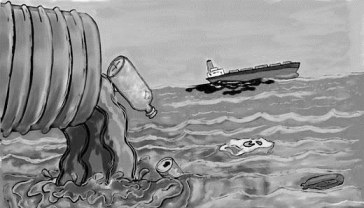1. Identify the type of pollution.

2. Name one pollutant that causes the above pollution.

3. Mention the impact of this pollution on human health.

4. State one measure to control this pollution.

5. What is a ‘Pollutant’? Explain the term.

### Section II (40 Marks)

Attempt any four questions from this Section.

Question 2

(a) Given below is an experimental setup to demonstrate a particular tropic movement in germinating seeds. Study the diagram and answer the questions that follow: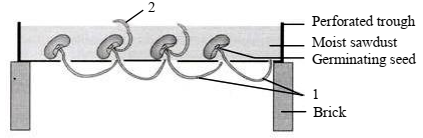1. Label the parts 1 and 2.

2. Name the tropic movement shown by part 1.

3. Part 1 is affected by two stimuli. Name them. Which one of the two is stronger?

4. What is Thigmotropism? Give one example.

5. What is meant by ‘Positive’ and ‘Negative’ tropic movements in plants?

(b) Mention the exact location of the following: 

1. Testis

2. Incus

3. Thylakoids

4. Amniotic fluid

5. Corpus callosum

Question 3

(a) The diagram given below represents an experiment to prove the importance of a factor in photosynthesis. Answer the questions that follow: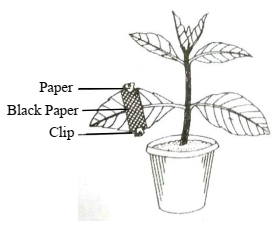1. Name the factor studied in this experiment.

2. What will you observe in the experimental leaf after the starch test?

3. Explain the process of Photosynthesis.

4. Give a balanced chemical equation to represent the process of photosynthesis.

5. Draw a neat, labelled diagram of an experimental setup to show that oxygen is released during photosynthesis.

(b) State the main functions of the following: 

1. Medulla Oblongata

2. Cytokinins

3. Tears

4. Coronary Artery

5. Seminal Vesicles

Question 4

(a) The diagram given below represents an organ system in the human body. Study the same and answer the questions that follow: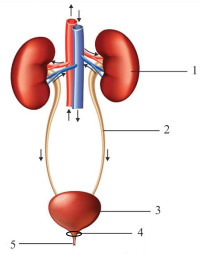1. Identify the system.

2. Label the parts marked 2 and 4. Mention the function of part 5.

3. Name the structural and functional units of the part marked 1.

4. What is the fluid that accumulates in part 3? Which is the main nitrogenous waste present in it?

5. Draw a neat, labelled diagram showing the longitudinal section of part 1.

(b) The diagram given below represents an endocrine gland in the human body. Study the diagram and answer the following questions: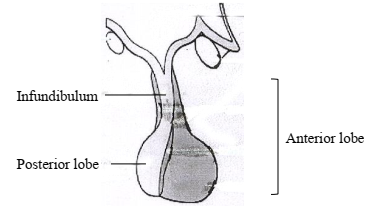1. Identify the endocrine gland. Where is it located?

2. Why is the above gland referred to as the ‘Master gland’?

3. Name the hormone which in deficiency causes Diabetes Insipidus. How does this disorder differ from Diabetes Mellitus?

4. Explain the term ‘Hormone’. What is the role of Tropic hormones in the human body?

5. Which lobe of the above gland secretes:

1. Oxytocin

2. ACTH

3. Growth hormone

Question 5

(a) Given below is an apparatus which was setup to investigate a physiological process in plants. The setup was placed in bright sunlight. Answer the questions that follow: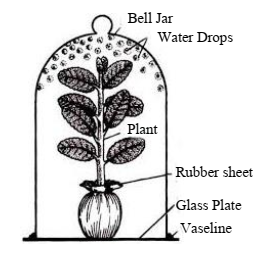1. Name the process being studied. Define the process.

2. Why was the pot enclosed in a rubber sheet?

3. Mention two external factors which can accelerate the above process.

4. List two adaptations in plants to reduce the above process.

5. Draw a neat, labelled diagram of a stomatal apparatus.

(b) Given below are two stages in the evolution of man. Study them and answer the questions that follow: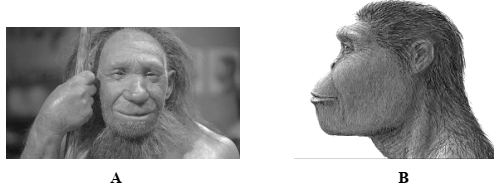1. Identify Australopithecus and Neanderthal man from the above pictures.

2. Mention two characteristic features each for the two stages.

3. Who proposed the theory of ‘Natural Selection’?

4. Name the organism used as an example to explain ‘Industrial Melanism’.

5. Give two examples of Vestigial organs in humans.

Question 6

(a) In Mendel’s experiments, tall pea plants (T) are dominant over dwarf pea plants (t). 

1. What is the phenotype and genotype of the F1 generation if a homozygous tall plant is crossed with a homozygous dwarf plant?

2. Draw a Punnett square board to show the gametes and offspring when both the parents are heterozygous for tallness.

3. What is the phenotypic ratio and genotypic ratio of the above cross in (ii)?

4. State Mendel’s Law of Dominance.

5. What is a Dihybrid Cross?

(b) Given below is a diagram representing a stage during the mitotic cell division. Study the diagram and answer the following questions: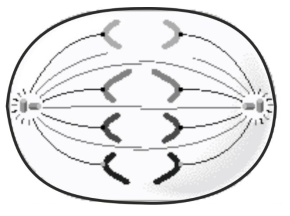1. Identify the stage by giving a suitable reason.

2. Is it a plant or an animal cell? Give a reason to support your answer.

3. Draw a neat, labelled diagram of the stage which follows the one shown in the diagram.

4. How many chromosomes will each daughter cell have after the completion of the above division?

5. Name the four nitrogenous bases.

Question 7

(a) Answer the following questions briefly: 

1. How are the cytons and axons placed in the brain and the spinal cord?

2. Which part of the human ear gives ‘Dynamic balance’ and ‘Static balance’ to the body?

3. Explain how the human eye adapts itself to bright light and dim light.

4. What is Parthenocarpy? Give one example.

5. Mention any two objectives of ‘Swachh Bharat Abhiyan’.

(b) The diagram given below represents a system in the human body. Study the diagram and answer the following questions: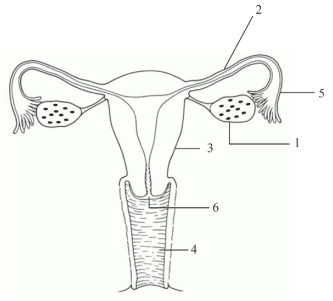1. Identify the system.

2. Label the parts marked 5 and 6.

3. Name the two hormones secreted by 1.

4. Mention the number and the name of the part involved in fertilization and implantation from the above diagram.

5. Mention the surgical methods of contraception in:

1. Human males.

2. Human females.